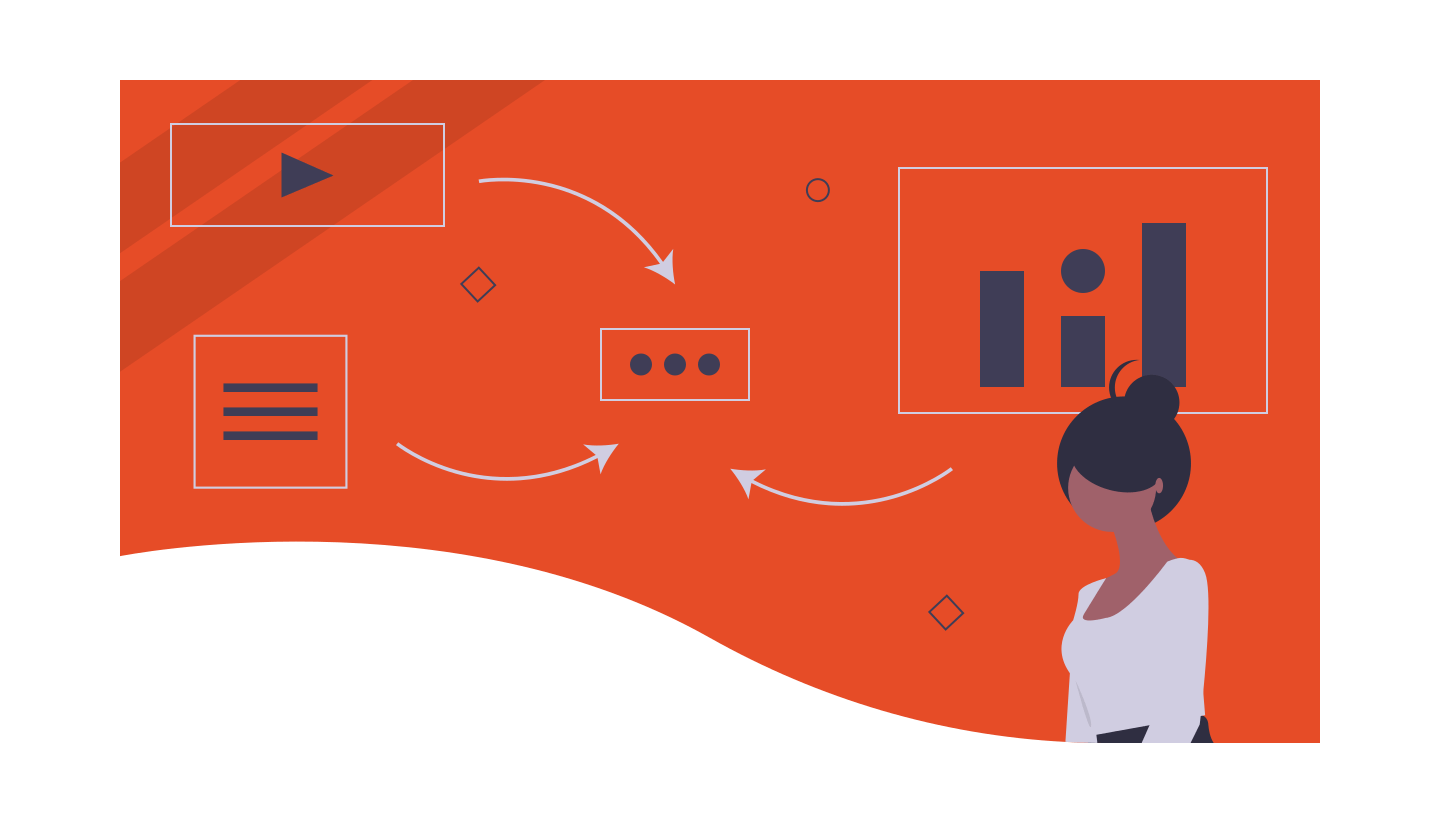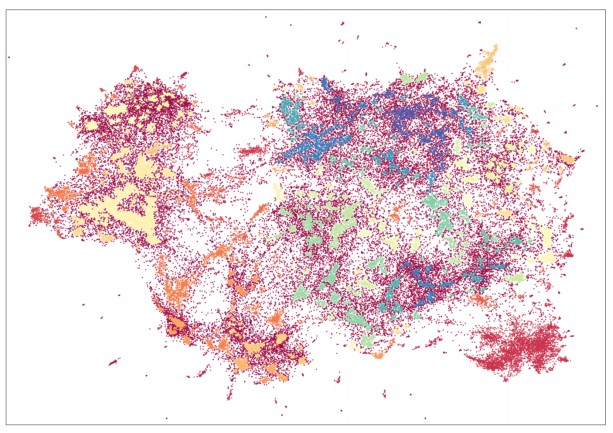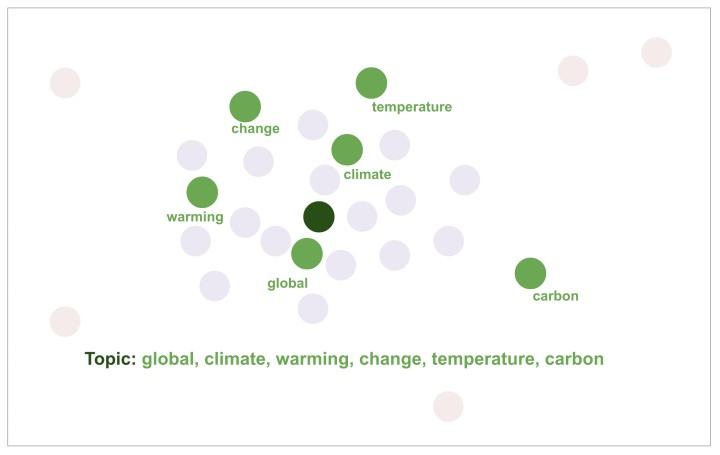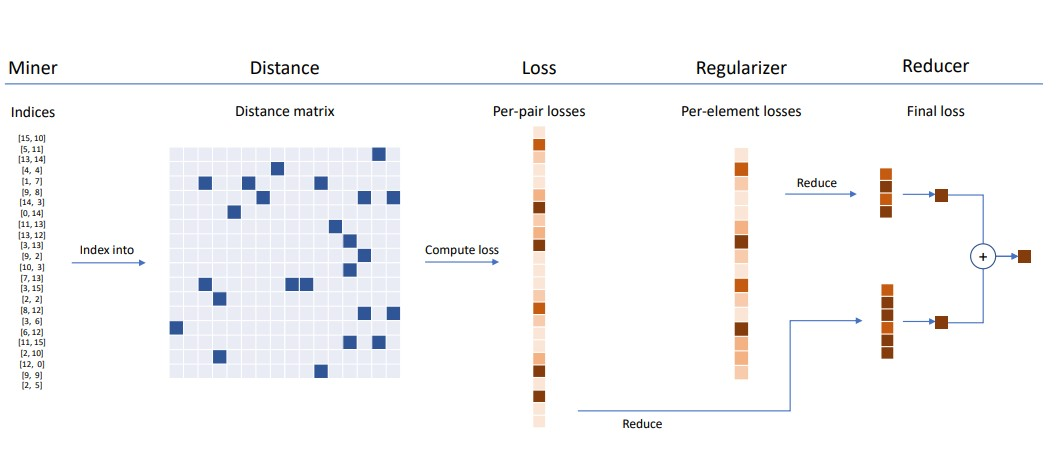Select Page

Every month, we decipher three research papers from the fields of machine learning, deep learning and artificial intelligence, which left an impact on us in the previous month. Apart from that, at the end of the article, we add links to other papers that we have found interesting but were not in our focus that month. So, you can check those as well. Here are the links from the previous months:

In general, we try to present papers that are going to leave a big impact on the future of machine learning and deep learning. We believe that these proposals are going to change the way we do our jobs and push the whole field forward. Have fun!

Are you afraid that AI might take your job? Make sure you are the one who is building it.

STAY RELEVANT IN THE RISING AI INDUSTRY! 🖖

## Top2Vec: Distributed Representation of Topics

One of the most interesting topics of NLP is organization and summarization of a large volume of text. The main method that is used to achieve this goal is called topic modeling. This approach discovers the latent semantic structure, or topics, present in the corpus of words (document). In general, the topic can be observed as a theme or a subject in the text. Sometimes they are discrete values, but often they are divided into sub-topics and overlap with each other. For example, politic and health topics can both be associated with health care sub-topic. Eventually, any of these topics, their combinations, or variations can be described by some unique set of weighted words.

One of the most used topics modeling method is Latent Dirichlet Allocation (LDA). It describes each document as a mixture of topics and each topic as a distribution of words. In essence, it separates continuous topic space into topics and models document as a mix of those topics. This is done so the relationship between document and words can be derived. However, this approach assumes that the number of topics is known beforehand, which is rarely the case. Another problem is the so-called stop words. These words are the ones that would have the highest frequency for each topic and are words that are used generally in the language (in English these would be words like ‘and’, ‘the’, etc.).In order to avoid this problem, LDA uses bag-of-words (BOW) representations of documents. This way word semantics are ignored. However, the problem with this is that in BOW representation the words Serbia and Serbian would be treated as different words, despite their semantic similarity. This is where stemming and lemmatization techniques come into play, further complicating the topic modeling approach.

That is why the authors of the paper propose another solution called top2vec. This solution is separated into three major steps:

• Creation of semantic embeddings
• Finding a number of topics
• Calculation of the topic vectors

In the first step, documents from the document set are clustered. Basically, by creating embeddings where the distance between document vectors and word vectors represents the semantic association, we cluster documents. Similar documents are joined together in the embedding space, and words are close to documents which they best describe. For this purpose, the doc2vec technique is used, which is similar to the word2vec technique. To be more precise doc2vec with Distributed Bag of Words (DBOW) is used. This model uses the document vector to predict words within a context window in the document.Once embeddings are available, this top2vec finds the number of topics. This is achieved by first lowering the dimension of embeddings with UMAP. Then the HDBSCAN with a minimum cluster size of 15 is used to find the dense areas of document vectors. Since HDBSCAN assigns a label to each dense cluster of document vectors and assigns a noise label to all document vectors that are not in a dense cluster. The number of dense clusters represents the number of topics.Finally, topic vectors are calculated by getting the centroid for each dense cluster that HDBSCAN detected. The word vectors that are closest to a topic vector are those that are most representative of it semantically. Since in this setup, the common words would appear in most of the documents, they would be far from all dense clusters, ie. further away from all topic vectors. This way stop words would be essentially marked as noise and no additional process for their removal is necessary. This is confirmed empirically.The code that accompanies this paper can be found here.

## PyTorch Metric Learning

Many solutions in machine learning require measuring the distance between some points. Even highly popular solutions, like recommendations systems or NLP approaches, rely on this very point. There are a variety of standard approaches that could be used for this purpose like Euclidean distance, City-Block distance, Cosine similarity, etc. However, often designing specific metrics for a specific problem is a task on its own. In fact, there is a whole sub-field of machine learning dedicated to that called – distance metrics learning or simply metric learning. This approach aims to automatically construct distance metric for a specific data using supervised data.

In essence, metric learning measures the similarity among samples while using an optimal distance metric for learning tasks. These methods are, however, limited in solving real-world problems demonstrating non-linear characteristics. In the past couple of years, deep metric learning has emerged and attracted some attention. In general, deep metrics learning provides a better solution for nonlinear data through activation functions, and thus it is better for solving non-linear problems. PyTorch Metric Learning is an open-source library that provides various metrics learning algorithms, whose implementation would otherwise be time-consuming. This library has 9 modules, displayed in the image below.These modules can be used separately or in combination for a complete train/test pipeline. Each of these modules has specific functionality. One of the most important ones is the Loss module which models loss functions. These work very much like regular PyTorch loss functions. However, as you can see in the image above its behavior can be modified and augmented with miners, distances, regularizers, and reducers. The whole process can be seen in the image below:In the first step, miners find the best samples which are used for training. This is an important concept in metric learning. There are two types of miners provided in this library:

• Online miners – find the best tuples within an already sampled batch
• Offline miners – which determine the best way to create batches

So, miners find the best pairs (since we are working with the 2D distance matrix) in the current batch. Pytorch Metric Learning library provides an easy way to use them:

``````from pytorch_metric_learning.losses import CircleLoss
from pytorch_metric_learning.miners import MultiSimilarityMiner
loss_func = CircleLoss()
mining_func = MultiSimilarityMiner()

embeddings = model(data)
hard_tuples = mining_func(embeddings, labels)
loss = loss_func(embeddings, labels, hard_tuples)
loss.backward()``````

These pars are used to index the distance matrix, which is modeled by the instance of distance class. The distance abstracts different types of distances (duh!). Loss function can be augmented with different distance objects which will change its behavior. For example, we might want our loss function to use Cosine Simmilarity instead of Euclidian Distance. Then we can do something like this:

``````from pytorch_metric_learning.losses import TripletMarginLoss
from pytorch_metric_learning.distances import CosineSimilarity
loss_func = TripletMarginLoss(distance = CosineSimilarity())``````

In a nutshell, the Loss function uses a distance object to compute a pairwise distance matrix and then uses elements of this matrix to compute the loss. Additionally, every loss function has an optional embedding regularizer parameter. This is a common thing to do in the process of metric learning, to add embedding or weight regularization terms to the loss. Regularization loss is computed for each embedding in the batch. Here is how you can do it with PyTorch Metric Learning library:

``````from pytorch_metric_learning.losses import ContrastiveLoss
from pytorch_metric_learning.regularizers import LpRegularizer
loss_func = ContrastiveLoss(embedding_regularizer = LpRegularizer())``````As you are aware, the loss is calculated for pairs or triplets and then reduced to a single value by some operation, such as averaging. Losses objects can receive a reduction parameter which defines how this operation will be performed. For example:

``````from pytorch_metric_learning.losses import MultiSimilarityLoss
from pytorch_metric_learning.reducers import ThresholdReducer
loss_func = MultiSimilarityLoss(reducer = ThresholdReducer(low = 10, high = 30))``````

Other modules in this library are there to cover some edge cases. For example, some metric learning algorithms are more than just losses or mining functions. Some algorithms require additional networks, data augmentations, learning rate schedules etc. That is what trainers are used for. Together with HookContainer class, you can turn trainers into a complete train/test workflow, with logging and model saving. Here is an example of the complete pipeline that can be used for metric learning on MNIST dataset:

``````from pytorch_metric_learning import losses, miners, distances, reducers, testers
from pytorch_metric_learning.utils.accuracy_calculator import AccuracyCalculator
### MNIST code originally from https://github.com/pytorch/examples/blob/master/mnist/main.py ###
from torchvision import datasets
import torch
import torch.nn as nn
import torch.nn.functional as F
import torch.optim as optim
from torchvision import datasets, transforms
import numpy as np

### MNIST code originally from https://github.com/pytorch/examples/blob/master/mnist/main.py ###
class Net(nn.Module):
def __init__(self):
super(Net, self).__init__()
self.conv1 = nn.Conv2d(1, 32, 3, 1)
self.conv2 = nn.Conv2d(32, 64, 3, 1)
self.dropout1 = nn.Dropout2d(0.25)
self.dropout2 = nn.Dropout2d(0.5)
self.fc1 = nn.Linear(9216, 128)

def forward(self, x):
x = self.conv1(x)
x = F.relu(x)
x = self.conv2(x)
x = F.relu(x)
x = F.max_pool2d(x, 2)
x = self.dropout1(x)
x = torch.flatten(x, 1)
x = self.fc1(x)
return x

### MNIST code originally from https://github.com/pytorch/examples/blob/master/mnist/main.py ###
def train(model, loss_func, mining_func, device, train_loader, optimizer, epoch):
model.train()
for batch_idx, (data, labels) in enumerate(train_loader):
data, labels = data.to(device), labels.to(device)
embeddings = model(data)
indices_tuple = mining_func(embeddings, labels)
loss = loss_func(embeddings, labels, indices_tuple)
loss.backward()
optimizer.step()
if batch_idx % 20 == 0:
print("Epoch {} Iteration {}: Loss = {}, Number of mined triplets = {}".format(epoch, batch_idx, loss, mining_func.num_triplets))

### convenient function from pytorch-metric-learning ###
def get_all_embeddings(dataset, model):
tester = testers.BaseTester()
return tester.get_all_embeddings(dataset, model)

### compute accuracy using AccuracyCalculator from pytorch-metric-learning ###
def test(dataset, model, accuracy_calculator):
embeddings, labels = get_all_embeddings(dataset, model)
print("Computing accuracy")
accuracies = accuracy_calculator.get_accuracy(embeddings,
embeddings,
np.squeeze(labels),
np.squeeze(labels),
True)
print("Test set accuracy (MAP@10) = {}".format(accuracies["mean_average_precision_at_r"]))

device = torch.device("cuda")

transform = transforms.Compose([
transforms.ToTensor(),
transforms.Normalize((0.1307,), (0.3081,))
])

batch_size = 256

dataset2 = datasets.MNIST('.', train=False, transform=transform)

model = Net().to(device)
num_epochs = 1

### pytorch-metric-learning stuff ###
distance = distances.CosineSimilarity()
reducer = reducers.ThresholdReducer(low = 0)
loss_func = losses.TripletMarginLoss(margin = 0.2, distance = distance, reducer = reducer)
mining_func = miners.TripletMarginMiner(margin = 0.2, distance = distance, type_of_triplets = "semihard")
accuracy_calculator = AccuracyCalculator(include = ("mean_average_precision_at_r",), k = 10)
### pytorch-metric-learning stuff ###

for epoch in range(1, num_epochs+1):
train(model, loss_func, mining_func, device, train_loader, optimizer, epoch)
test(dataset2, model, accuracy_calculator)``````

The code that accompanies this paper can be found here.

## AP-Loss for Accurate One-Stage Object Detection

If you work in computer vision, you probably know that object detection is an important topic with a lot of solutions flying around. Especially the new ones which use deep learning. In general, these solutions are separated into two types: one-stage detectors and two-stage detectors. The main difference is that two-stage detector a number of object box proposals and then perform classification and localization tasks on them, while one-stage detectors predict the object class directly from the pre-designed candidate boxes – anchors. This difference causes one-stage detectors to be faster, but two-stage detectors to have higher accuracy.

One possible reason for the one-stage problems with accuracy lies in the extreme imbalance between foreground and background regions, which causes class bias during optimization of the classification task. In order to address this issue, some studies and solutions proposed new classification losses. These losses model each anchor box independently and attempt to re-weight the foreground and background samples within the classification loss to cater for the imbalanced condition.

However, because they ignore the relationship of the anchor boxes it is hard to distinguish important anchor boxes from non-important ones. This makes these options limited. That is why authors of this paper, instead of changing the classification loss, propose to tackle this problem as a ranking task with AP (Average Precision) ranking metric. In object detection, the AP metric evaluates detection results by considering both precision and recall at different thresholds. However, since it is non-differentiable, optimization of this loss is quite a challenge, since regular techniques like Stochastic Gradient Descent can not be used. So, this paper proposed a novel error-driven learning algorithm to effectively optimize the non-differentiable AP-based objective function.In the image above, the complete framework is presented. We can see that classification-task in one-stage detectors is replaced with a ranking task. The ranking
procedure produces the primary terms of AP-loss and the corresponding label vector. Finally, the optimization algorithm is based on an error-driven learning scheme combined with backpropagation. This “error-driven learning algorithm” is generalized from the perceptron learning algorithm which is how it helps overcome the difficulty of the non-differentiable objective functions. This means that the update is derived from the difference between the desired output and current output. The solution is quite elegant.

The beauty of the ranking task is that instead of the calculating label with IOU, like a traditional one-stage detector would do, it replicates each anchor box for each class and then calculates labels for each replicated box again using IOU. This label is then coming from set {-1, 0, 1} where -1 indicates this anchor is ignored, 0 indicates this is the background, and 1 indicates that this belongs to the class for which this replicated anchor box is created. The ranking task dictates that every positive box should be ranked higher than all the negative boxes. This enables an easier calculation of AP-loss.

The code that accompanies this paper can be found here.

## Conclusion

In this article, we had a chance to real about three really cool papers that, as we see it, are going to change the way we perform our jobs. Did you have any favorites this month? Let us know.#### Nikola M. Zivkovic

CAIO at Rubik's Code

Nikola M. Zivkovic a CAIO at Rubik’s Code and the author of book “Deep Learning for Programmers“. He is loves knowledge sharing, and he is experienced speaker. You can find him speaking at meetups, conferences and as a guest lecturer at the University of Novi Sad.

Rubik’s Code is a boutique data science and software service company with more than 10 years of experience in Machine Learning, Artificial Intelligence & Software development. Check out the services we provide.

Ultimate Guide to Machine Learning with Python

Everything from Python basics to the deployment of Machine Learning algorithms to production in one place.

Become a Machine Learning Superhero TODAY!